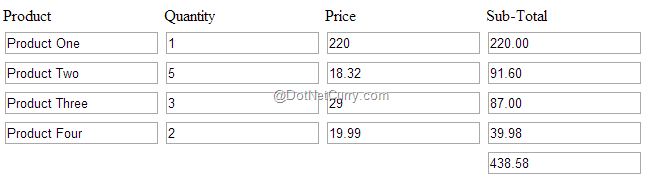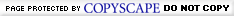# Using jQuery to Perform Calculations in a Table

Posted by: Suprotim Agarwal , on 9/27/2015, in Category jQuery and ASP.NET
Views: 107879
Abstract: Use jQuery to traverse all of the values in a HTML table column, convert the values to numbers, and then sum the values.

JavaScript supports basic mathematical operations like addition, subtraction, division and so on. These mathematical operations makes sense on numbers, but in HTML, the data that we read is in string format, which means the data is to converted to a number, before we can perform mathematical operations on them. In this article, we will learn how to use jQuery to traverse all of the values in a HTML table column, convert the values to numbers, and then sum the values.

Create a new file called ‘TableCalculateTotal.html’ in a folder. We will need a simple HTML Table to get started. Our table has an id attribute of tblProducts and a thead , tbody and tfoot to go with it.

```<table id="tblProducts">
...
<tbody>
...
</tbody>
<tfoot>
...
</tfoot>
</table>

```

The Table has 4 columns – Product, Quantity, Price and Sub-Total. It is assumed here that the Product and the Price info will be prepopulated (in your case probably from a database). When the user enters the Quantity, the Sub-Total is automatically calculated using Price x Quantity. The <tfoot> contains a row representing a GrandTotal which is the sum of all the cells in the Sub-Total column.

```<thead>
<tr>
<td>Product</td>
<td>Quantity</td>
<td>Price</td>
<td>Sub-Total</td>
</tr>
<tbody>
<tr>
<td><input type="text" class="pnm" value="Product One" name="pnm" /></td>
<td><input type="text" class="qty" value="" name="qty"/></td>
<td><input type="text" class="price" value="220" name="price"/></td>
<td><input type="text" class="subtot" value="0" name="subtot"/></td>
</tr>
</tbody>
<tfoot>
<tr>
<td></td>
<td></td>
<td></td>
<td><input type="text" class="grdtot" value="" name=""/></td>
</tr>
</tfoot>
</table>

```

Let’s now see the script which will calculate the Sub-Total column values. We will also sum all of the values in the Sub-Total column, and display the result in the table footer.

The following script selects all of the table rows <tr>’s within the table body. The next step is to use jQuery's built-in each() iterator to loop over this collection of ‘tr’ elements. For each iteration of the loop, \$(this) refers to a ‘tr’ element, which is being assigned to a local variable \$row.

```var \$tblrows = \$("#tblProducts tbody tr");
\$tblrows.each(function (index) {
var \$tblrow = \$(this);

...
});

```

We are using a well-structured markup here with a ‘thead’ and ‘tbody’ so we could use \$("#tblProd tbody tr") to select all rows in the table body. If you do not have a thead, tbody and instead your first row is a header row, then use this selector to skip the first row:

```var \$tblrows = \$("#tblProducts tr:gt(0)");
```

Every time the user enters a value in the Quantity field, the subtotal column should be automatically populated by multiplying the Price with the Quantity entered. The following script achieves this functionality:

```\$tblrow.find('.qty').on('change', function () {

var qty = \$tblrow.find("[name=qty]").val();
var price = \$tblrow.find("[name=price]").val();
var subTotal = parseInt(qty,10) * parseFloat(price);

...
});

```

Both global functions parseInt and parseFloat convert strings to numbers. I tend to use parseFloat over parseInt, as it is more adaptable in scenarios where I am unsure if all of the numbers will be integers. parseFloat works with both integers and floating-point numbers. In this example, I am assuming that the values for Quantity are coming from my database, so they do not contain any decimals. In such scenarios, I can safely use parseInt for integer columns.

If you observe, the parseInt function has two arguments: a required numeric string, and an optional radix (base). The radix is the number’s base, as in base-8 (octal), base-10 (decimal) and base-16 (hexadecimal). If the radix is not provided, it’s assumed to be 10, for decimal. Although the second argument is optional, it’s considered a good practice to always provide it explicitly.

If the string provided doesn’t contain a number, NaN is returned. So we should check to see if the subTotal is not a NaN. The next step is to use toFixed() method to format the subTotal to two decimal points.

```if (!isNaN(subTotal)) {

\$tblrow.find('.subtot').val(subTotal.toFixed(2));
...
}

```

We then use each() to loop through the subTotal column and sum the text of subtotal in each row and assign the result to the grandTotal variable. The last step is to assign the result to the grandTotal cell.

```if (!isNaN(subTotal)) {

\$tblrow.find('.subtot').val(subTotal.toFixed(2));
var grandTotal = 0;

\$(".subtot").each(function () {
var stval = parseFloat(\$(this).val());
grandTotal += isNaN(stval) ? 0 : stval;
});

\$('.grdtot').val(grandTotal.toFixed(2));
}

```

Save and load the page in your browser, enter the Quantity and you should see the Sub-Total rows populated, as well as a sum of the Sub-Total in the footer of your table.C# and .NET have been around for a very long time, but their constant growth means there’s always more to learn.

We at DotNetCurry are very excited to announce The Absolutely Awesome Book on C# and .NET. This is a 500 pages concise technical eBook available in PDF, ePub (iPad), and Mobi (Kindle).

Organized around concepts, this Book aims to provide a concise, yet solid foundation in C# and .NET, covering C# 6.0, C# 7.0 and .NET Core, with chapters on the latest .NET Core 3.0, .NET Standard and C# 8.0 (final release) too. Use these concepts to deepen your existing knowledge of C# and .NET, to have a solid grasp of the latest in C# and .NET OR to crack your next .NET Interview.

AuthorSuprotim Agarwal, MCSD, MCAD, MCDBA, MCSE, is the founder of DotNetCurry, DNC Magazine for Developers, SQLServerCurry and DevCurry. He has also authored a couple of books 51 Recipes using jQuery with ASP.NET Controls and The Absolutely Awesome jQuery CookBook.

Suprotim has received the prestigious Microsoft MVP award for Fifteen consecutive years. In a professional capacity, he is the CEO of A2Z Knowledge Visuals Pvt Ltd, a digital group that offers Digital Marketing and Branding services to businesses, both in a start-up and enterprise environment.Feedback - Leave us some adulation, criticism and everything in between!